Tamilnadu State Board New Syllabus Samacheer Kalvi 12th Maths Guide Pdf Chapter 6 Applications of Vector Algebra Ex 6.7 Textbook Questions and Answers, Notes.

## Tamilnadu Samacheer Kalvi 12th Maths Solutions Chapter 6 Applications of Vector Algebra Ex 6.7

Question 1.
Find the non-parametric form of vector equation and Cartesian equation of the plane passing through the point (2, 3, 6) and parallel to the .straight lines.
$$\frac { x-1 }{ 2 }$$ = $$\frac { y+1 }{ 3 }$$ = $$\frac { z-3 }{ 1 }$$ and $$\frac { x+3 }{ 2 }$$ = $$\frac { y-3 }{ -5 }$$ = $$\frac { z+1 }{ -3 }$$
Solution: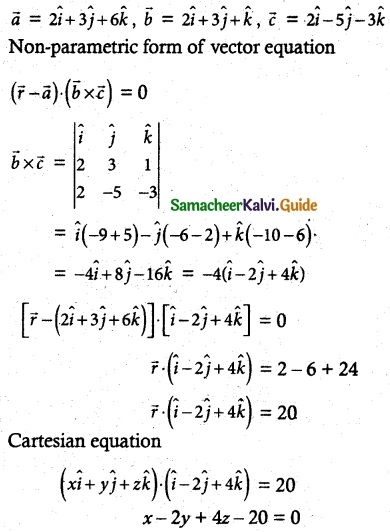Question 2.
Find the non-parametric form of vector equation and Cartesian equations of the plane passing through the points (2, 2, 1), (9, 3, 6) and perpendicular to the plane 2x + 6y + 6z = 9.
Solution: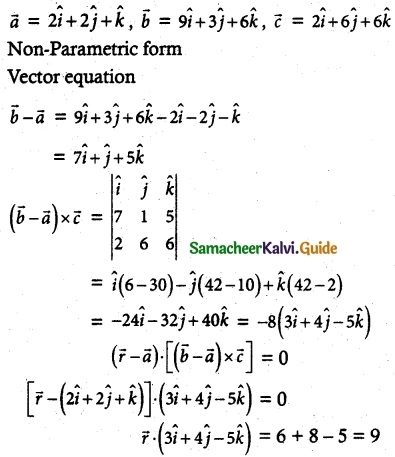Cartesian equation
3x + 4y – 5z = 9
3x + 4y – 5z – 9 = 0

Question 3.
Find parametric form of vector equation and Cartesian equations of the plane passing through the points (2, 2, 1), (1, -2, 3) and parallel to the straight line passing through the points (2, 1, -3) and (-1, 5, -8)
Solution: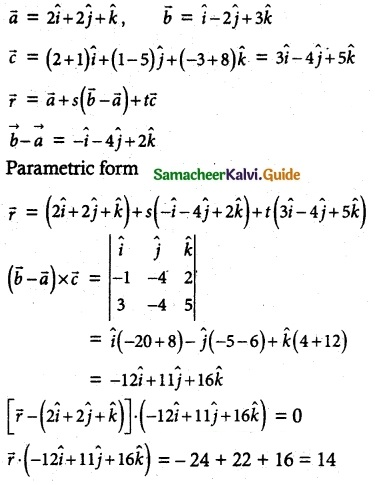Cartesian equation
-12x + 11y + 16z = 14
12x – 11y – 16z = -14
12x – 11y – 16z + 14 = 0Question 4.
Find the non-parametric form of vector equation and Cartesian equation of the plane passing through the point (1, -2, 4) and perpendicular to the plane x + 2y – 3z = 11 and parallel to the line $$\frac { x+7 }{ 3 }$$ = $$\frac { y+3 }{ -1 }$$ = $$\frac { z }{ 1 }$$
Solution: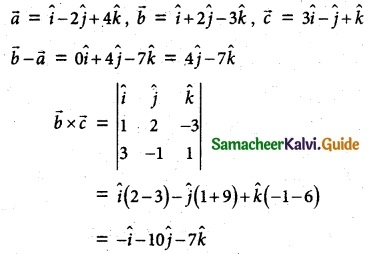Non parametric form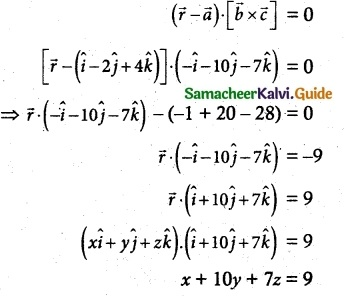Which is the required Cartesian equation of the place.

Question 5.
Find the parametric form of vector equation and Cartesian equations of the plane containing
the line $$\overline { r }$$ = ($$\hat { i }$$ – $$\hat { j }$$ + 3$$\hat { k }$$) + t(2$$\hat { i }$$ – $$\hat { j }$$ + 4$$\hat { k }$$ ) and perpendicular to plane $$\overline { r }$$ ($$\hat { i }$$ + 2$$\hat { j }$$ + $$\hat { k }$$) = 8
Solution: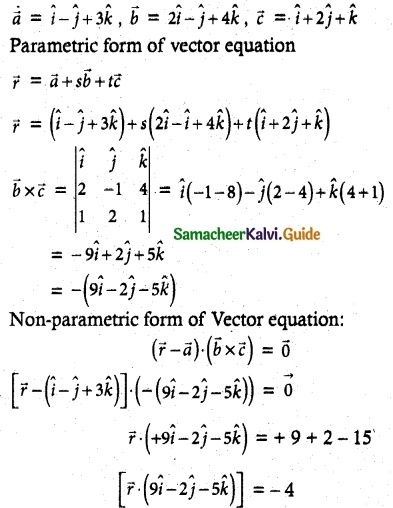Cartesian equation
9x – 2y – 5z = -4
9x – 2y – 5z + 4 = 0Question 6.
Find the parametric vector non-parametric vector and Cartesian form of the equations of the plane passing through the three non- collinear points (3, 6, -2), (-1, -2, 6) and (6, 4, -2).
Solution: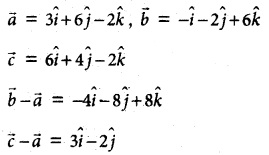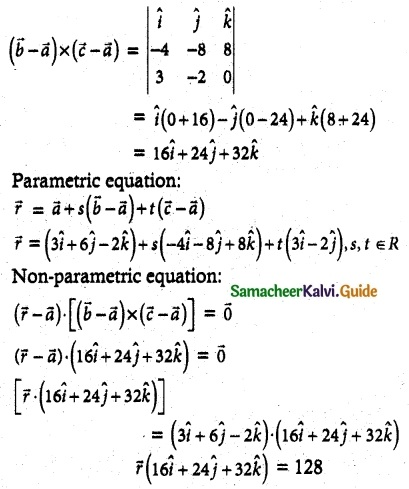Cartesian equation
⇒ $$\overline { r }$$(2$$\hat { i }$$ + 3$$\hat { j }$$ + 4$$\hat { k }$$) = 16
⇒ 2x + 3y + 4z – 16 = 0

Question 7.
Find the non-parametric form of vector equation and Cartesian equations of the plane
$$\overline { r }$$ = (6$$\hat { i }$$ – $$\hat { j }$$ + $$\hat { k }$$) + s($$\hat { -i }$$ + 2$$\hat { j }$$ + $$\hat { k }$$) + t($$\hat { -5i }$$ – 4$$\hat { j }$$ – 5$$\hat { k }$$)
Solution: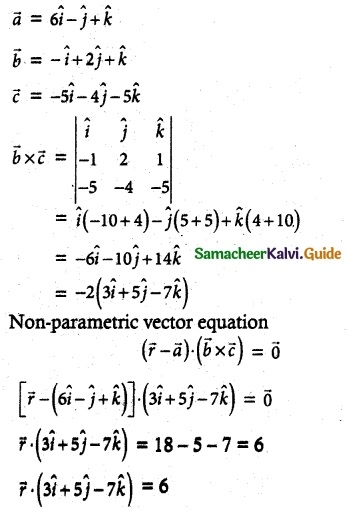Cartesian equation:
3x + Sy – 7z = 6
3x + 5y – 7z – 6 = 0